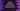# Python program to insert multiple elements to a list at any specific position## Insert multiple elements to a list using python :

In this python programming tutorial, we will learn how to insert multiple elements to a list at a specific position.

The list is given to the user and the program will ask the user to enter the values to insert in the list at any specific position. Using a loop, it will read the values and insert them in that position of the list.

## Algorithm :

The algorithm of the program is like below :

1. The original list is given to the user.
2. Ask the user to enter the index to insert the new elements.
3. Run one loop to take the inputs from the user one by one.
4. Insert the elements at the index user has mentioned in step 2.
5. Finally, print the final list.

For example, if the list is [8,9,10,11,12] and if we want to insert_ [‘a’,‘b’]_ in index 2, it will look like as below :

Step 1 : Insert ‘a’ to index 2 : [8,9,‘a’,10,11,12]. Step 2 : Insert ‘b’ to index 3 : [8,9,‘a’,‘b’,10,11,12]. Step 3 : Final list :_ [8,9,‘a’,‘b’,10,11,12]._

## Python program :

The python looks like below :

``````#1
user_list = ['a', 'b', 'c', 'd', 'e']

#2
print("Original list : {}".format(user_list))

#3
count = int(input("Enter the total number of elements to add : "))
index = int(input("Enter the index in the list : "))

#4
for i in range(count):
#5
user_input_value = int(input("Enter element {} : ".format(i)))
user_list.insert(index+i, user_input_value)

#6
print("Final list : {}".format(user_list))``````

This program is also available in [Github](https://github.com/codevscolor/codevscolor/blob/master/python/insertmultipleelementslist.py)._

### Explanation :

The commented numbers in the above program denote the step numbers below :

1. userlist_ is the given list.
2. Print the original list to the user.
3. Get the_ total number_ to add to the list from the user as input.
4. Run one for loop to get the values from the user to insert in the list.
5. Read the user input and store it in userinputvalue. Insert this value to the list.
6. Print the final list to the user.

### Output :

``````Original list : ['a', 'b', 'c', 'd', 'e']
Enter the total number of elements to add : 3
Enter the index in the list : 2
Enter element 0 : 1
Enter element 1 : 2
Enter element 2 : 3
Final list : ['a', 'b', 1, 2, 3, 'c', 'd', 'e']``````